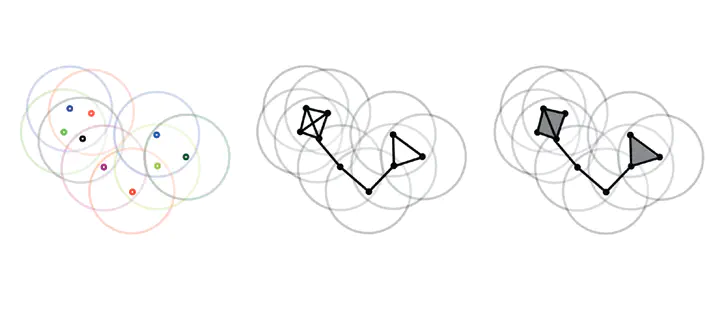# Computing discrete Morse complexes from simplicial complexes### Abstract

We consider the problem of efficiently computing a discrete Morse complex on simplicial complexes of arbitrary dimension and very large size. Based on a common graph-based formalism, we analyze existing data structures for simplicial complexes, and we define an efficient encoding for the discrete Morse gradient on the most compact of such representations. We theoretically compare methods based on reductions and coreductions for computing a discrete Morse gradient, proving that the combination of reductions and coreductions produces new mutually equivalent approaches. We design and implement a new algorithm for computing a discrete Morse complex on simplicial complexes. We show that our approach scales very well with the size and the dimension of the simplicial complex also through comparisons with the only existing public-domain algorithm for discrete Morse complex computation. We discuss applications to the computation of multi-parameter persistent homology and of extremum graphs for visualization of time-varying 3D scalar fields.

Type
Publication
Graphical Models